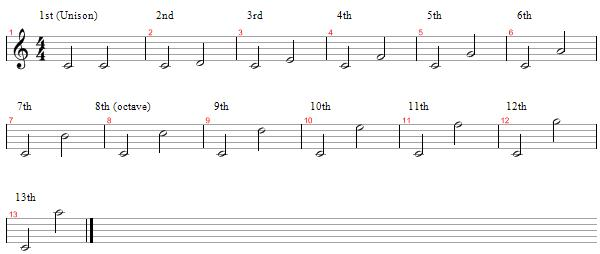• Lessons
• Articles

# Intervals Pt.1 (Intro - Major and Perfect Intervals)

Robert Mussatti 91 lessons

Before any of you start to delve into chords, scales, or arpeggios, you must first understand the basis of what and how intervals are formed. In a nutshell, an interval is the distance between any two notes.

There are only two types of intervals: harmonic and melodic. A harmonic interval is two notes that are played at the same time, and a melodic interval are two notes that are played at the same time like a melody. Intervals also have 2 attributes as well: size and quality. In order to determine its quality, you must figure out the size of the interval.

The size of an interval is very easy to figure out. Just count up the number of lines and spaces on the staff from the lowest note to the next note, but remember to count the starting note as 1. So for example, from C to E would not be 2, it would be 3 because we are counting the C as 1.

You are also going to encounter another grouping of intervals which are simple and compound. A simple interval are the ones found within the musical octave, and a compound interval are the ones found beyond the octave, which are from the 9th all the way to the 13th. The chart below shows the list of intervals you are going to encounter all the way up to the 13th.The first 2 intervals we will go through are Perfect and Major intervals. The Perfect intervals are the Unison, 4th, 5th, 8th, 11th and 12th. The Major intervals are on the 2nd, 3rd, 6th, 7th, 9th and 13th. Remember that the 9th is the same as the second, the 11th is the same as the 4th, the 12th is the same as the 5th, and the 13th is the same as the 6th.

This concludes our first lesson on intervals. In part 2, we will go over minor, diminished and augmented intervals.

Send this to a friend